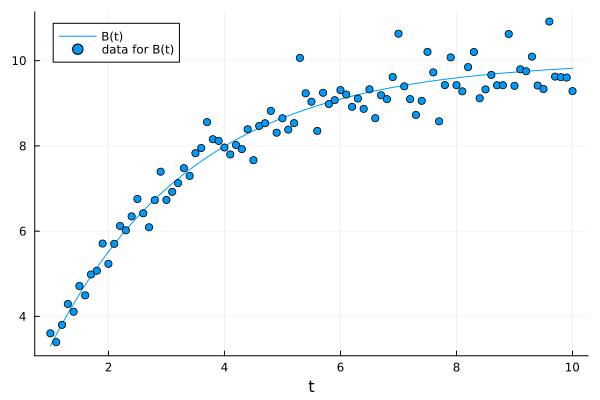# Custom Loss Functions

In this tutorial, we describe how to use custom loss functions withim PumasQSP workflows. By default PumasQSP uses the l2loss, but this is not always the best choice. This tutorial assumes that you have read the getting started tutorial.

## Julia environment

For this tutorial we will need the following packages:

ModuleDescription
PumasQSPPumasQSP is used to formulate the QSP workflow.
CatalystSymbolic modeling package for simulation of chemical reaction networks leveraging ModelingToolkit.
OrdinaryDiffEq (DifferentialEquations.jl)Numerical differential equation solvers
DataSetsWe will load our experimental data from datasets on JuliaHub.
PlotsThe plotting and visualization library used for this tutorial.

First we prepare the environment by listing the packages we are using within the example.

using PumasQSP
using Catalyst
using OrdinaryDiffEq
using DataSets
using Plots

## Model setup

We use the same model as the getting started tutorial.

# Model definition
rn = @reaction_network begin
@species A(t) = 10.0 B(t) = 0.0
@parameters k = 0.5
k, A --> B
end
model  = convert(ODESystem, rn)
\begin{align} \frac{\mathrm{d} A\left( t \right)}{\mathrm{d}t} =& - k A\left( t \right) \\ \frac{\mathrm{d} B\left( t \right)}{\mathrm{d}t} =& k A\left( t \right) \end{align}

However, the data is noisier and the strength of the noise seems to depend on the amount of species $B$. The data can be read in using the dataset function. Note that if you are using PumasQSP locally, you might have to follow these steps to make the dataset available.

data = dataset("pumasqsptutorials/reaction_data_heterogeneous")
name = "pumasqsptutorials/reaction_data_heterogeneous"
uuid = "2d935727-070e-4a89-a869-486360041d16"
description = "This file contains simulation data of the custom loss tutorial."
type = "Blob"

[storage]
driver = "JuliaHubDataRepo"
bucket_region = "us-east-1"
bucket = "internaljuilahubdatasets"
credentials_url = "https://internal.juliahub.com/datasets/2d935727-070e-4a89-a869-486360041d16/credentials"
prefix = "datasets"
vendor = "aws"
type = "Blob"

[storage.juliahub_credentials]
auth_toml_path = "/home/github_actions/actions-runner-1/home/.julia/servers/internal.juliahub.com/auth.toml"

[[storage.versions]]
blobstore_path = "u1"
date = "2023-08-15T20:18:12.744+00:00"
size = 2009
version = 1


For heteroscedastic noise a weighted variant of l2loss outperforms the default l2loss. Specifically, we assume that the standard deviation of the measurement noise increases linearly as B increases. We can implement such a weighted loss ourselves using the err keyword of trial. err should always be a function with two inputs, the solution of the model, at a certain point in the search space, and the data. Here the solution will always only contain the states and timepoints present in the dataset, e.g. state A will not be present in the solution. The dataset is transformed from a DataFrame to a Matrix. Both inputs are ordered in the same way, i.e. solution[i,j] corresponds to the same state and timepoint as dataset[i,j]. We can then easily implement losses using broadcasting.

function weighted_loss(solution, dataset)
sum(((solution .- dataset)./dataset).^2)
end
trial = Trial(data, model; err = weighted_loss)
@unpack k = model
search_space = [k => (0.1, 10.0)]
invprob = InverseProblem(trial, model, search_space)
alg = SingleShooting(maxiters = 10^4)
r = calibrate(invprob, alg)
Calibration result computed in 23 seconds and 198 milliseconds. Final objective value: 0.197286.
Optimization ended with Success Return Code and returned.

┌──────────┐
│        k │
├──────────┤
│ 0.401077 │
└──────────┘


We visualize results and see that a model simulation based on the calibrated reaction rate fits the data well.

plot(trial, invprob, r, show_data = true, legend = true)